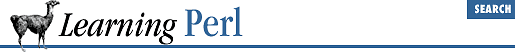home | O'Reilly's CD bookshelfs | FreeBSD | Linux | Cisco | Cisco Exam

### 4.2 The if/unless Statement

Next up in order of complexity is the ``` if``` statement. This construct takes a control expression (evaluated for its truth) and a block. It may optionally have an else followed by a block as well. In other words, it looks like this:

```if (```

some_expression
```
) {
```

true_statement_1
```
;
```

true_statement_2
```
;
```

true_statement_3
```
;
}

else {
```

false_statement_1
```
;
```

false_statement_2
```
;
```

false_statement_3
```
;
}```

(If you're a C or Java hacker, you should note that the curly braces are required. This eliminates the need for a " confusing dangling else" rule.)

During execution, Perl evaluates the control expression. If the expression is true, the first block (the ``` true_statement ``` statements above) is executed. If the expression is false, the second block (the ``` false_statement ``` statements above) is executed instead.

But what constitutes true and false? In Perl, the rules are slightly weird, but they give you the expected results. The control expression is evaluated for a string value in scalar context (if it's already a string, no change, but if it's a number, it is converted to a string[ 1 ]). If this string is either the empty string (with a length of zero), or a string consisting of the single character ``` "0"``` (the digit zero), then the value of the expression is false. Anything else is true automatically. Why such funny rules? Because it makes it easy to branch on an emptyish versus nonempty string, as well as a zero versus nonzero number, without having to create two versions of interpreting true and false values. Here are some examples of true and false interpretations:

```0       # converts to "0", so false
1-1     # computes to 0, then converts to "0", so false
1       # converts to "1", so true
""      # empty string, so false
"1"     # not "" or "0", so true
"00"    # not "" or "0", so true (this is weird, watch out)
"0.000" # also true for the same reason and warning
undef   # evaluates to "", so false```

 Internally, this isn't quite true. But it acts like this is what it does.

Practically speaking, interpretation of values as true or false is fairly intuitive. Don't let us scare you.

Here's an example of a complete ``` if``` statement:

```print "how old are you? ";
\$a = <STDIN>;
chomp(\$a);
if (\$a < 18) {
print "So, you're not old enough to vote, eh?\n";
} else {
print "Old enough!  Cool!  So go vote!\n";
\$voter++; # count the voters for later
}```

You can omit the ``` else``` block, leaving just a "then" part, as in:

```print "how old are you? ";
\$a = <STDIN>;
chomp(\$a);
if (\$a < 18) {
print "So, you're not old enough to vote, eh?\n";
}```

Sometimes, you want to leave off the "then" part and have just an ``` else``` part, because it is more natural to say "do that if this is false," rather than "do that if not this is true." Perl handles this with the ``` unless``` variation:

```print "how old are you? ";
\$a = <STDIN>;
chomp(\$a);
unless (\$a < 18) {
print "Old enough!  Cool!  So go vote!\n";
\$voter++;
}```

Replacing ``` if``` with ``` unless``` is in effect saying "If the control expression is false, do...." (An ``` unless``` can also have an ``` else``` , just like an ``` if``` .)

If you have more than two possible choices, add an ``` elsif``` branch to the ``` if``` statement, like so:

```if (```

some_expression_one
```
) {
```

one_true_statement_1
```
;
```

one_true_statement_2
```
;
```

one_true_statement_3
```
;
}

elsif (```

some_expression_two
```
) {
```

two_true_statement_1
```
;
```

two_true_statement_2
```
;
```

two_true_statement_3
```
;
} elsif (```

some_expression_three
```
) {
```

three_true_statement_1
```
;
```

three_true_statement_2
```
;
```

three_true_statement_3
```
;
} else {
```

all_false_statement_1
```
;
```

all_false_statement_2
```
;
```

all_false_statement_3
```
;
}```

Each expression (here, ``` some_expression_one ``` , ``` some_expression_two ``` , and ``` some_expression_three ``` ) is computed in turn. If an expression is true, the corresponding branch is executed, and all remaining control expressions and corresponding statement blocks are skipped. If all expressions are false, the ``` else``` branch is executed (if there is one). You don't have to have an ``` else``` block, but it is always a good idea. You may have as many ``` elsif``` branches as you wish.4.1 Statement Blocks4.3 The while/until Statement